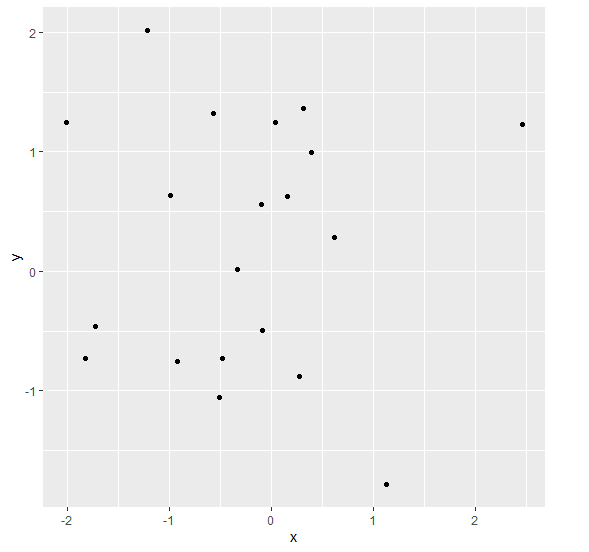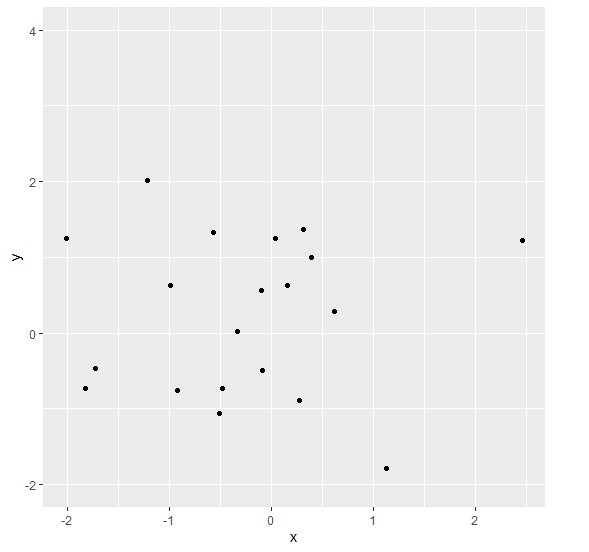# How to increase the length of Y-axis values for ggplot2 graph in R?

R ProgrammingServer Side ProgrammingProgramming

To increase the length of Y-axis for ggplot2 graph in R, we can use scale_y_continuous function with limits argument.

For Example, if we have a data frame called df that contains two columns say X and Y and we want to have the length of Y-axis starting from 1 to 10 by using the below mentioned command −

ggplot(df,aes(X,Y))+geom_point()+scale_y_continuous(limits=c(1,10))

## Example

Following snippet creates a sample data frame −

x<-rnorm(20)
y<-rnorm(20)
df<-data.frame(x,y)
df

The following dataframe is created

            x           y
1 -2.01096346  1.24463902
2  0.39496846  0.99334822
3 -0.51097047 -1.05482740
4  0.03373439  1.24457996
5  1.12358986 -1.78640864
6 -0.92102967 -0.75407431
7  0.31621086  1.36239416
8 -0.09043105 -0.49680298
9 -0.09693602  0.55751140
10 -1.21945066  2.01970155
11 -0.99525818  0.63536276
12 -0.57271775  1.32510316
13  0.15420177  0.62855015
14 -0.47752137 -0.73269498
15 -1.72463693 -0.46193220
16 -0.33377801  0.01744036
17 0.27385681 -0.88326612
18 0.61387778 0.28415277
19 -1.82064454 -0.73278798
20 2.46056155 1.22580050

To load ggplot2 package and create scatterplot between x and y on the above created data frame, add the following code to the above snippet −

x<-rnorm(20)
y<-rnorm(20)
df<-data.frame(x,y)
library(ggplot2)
ggplot(df,aes(x,y))+geom_point()

## Output

If you execute all the above given snippets as a single program, it generates the following Output −To create scatterplot between x and y with y values starting from -2 and ending at 4 on the above created data frame, add the following code to the above snippet −

x<-rnorm(20)
y<-rnorm(20)
df<-data.frame(x,y)
library(ggplot2)
ggplot(df,aes(x,y))+geom_point()+scale_y_continuous(limits=c(-2,4))

## Output

If you execute all the above given snippets as a single program, it generates the following Output −